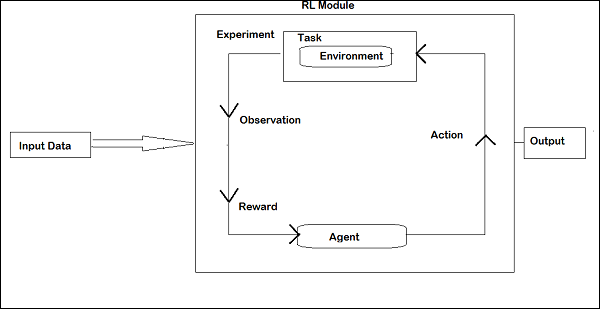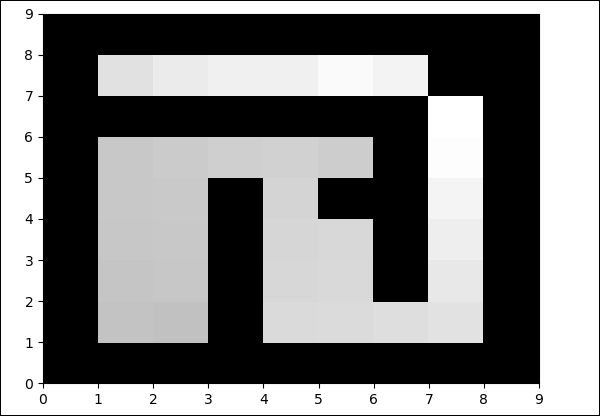# PyBrain - Reinforcement Learning Module

Reinforcement Learning (RL) is an important part in Machine Learning. Reinforcement learning makes the agent learn its behaviour based on inputs from the environment.

The components that interact with each other during Reinforcement are as follows −

• Environment
• Agent
• Experiment

The layout of Reinforcement Learning is given below −In RL, the agent talks with the environment in iteration. At each iteration, the agent receives an observation which has the reward. It then chooses the action and sends to the environment. The environment at each iteration moves to a new state and the reward received each time is saved.

The goal of RL agent is to collect as many rewards as possible. In between the iteration the agent's performance is compared with that of the agent that acts in a good way and the difference in performance gives rise to either reward or failure. RL is basically used in problem solving tasks like robot control, elevator, telecommunications, games etc.

Let us take a look at how to work with RL in Pybrain.

We are going to work on maze environment which will be represented using 2 dimensional numpy array where 1 is a wall and 0 is a free field. The agent's responsibility is to move over the free field and find the goal point.

Here is a step by step flow of working with maze environment.

## Step 1

Import the packages we need with the below code −

from scipy import *
import sys, time
import matplotlib.pyplot as pylab # for visualization we are using mathplotlib

from pybrain.rl.learners.valuebased import ActionValueTable
from pybrain.rl.agents import LearningAgent
from pybrain.rl.learners import Q, QLambda, SARSA #@UnusedImport
from pybrain.rl.explorers import BoltzmannExplorer #@UnusedImport
from pybrain.rl.experiments import Experiment


## Step 2

Create the maze environment using the below code −

# create the maze with walls as 1 and 0 is a free field
mazearray = array(
[[1, 1, 1, 1, 1, 1, 1, 1, 1],
[1, 0, 0, 1, 0, 0, 0, 0, 1],
[1, 0, 0, 1, 0, 0, 1, 0, 1],
[1, 0, 0, 1, 0, 0, 1, 0, 1],
[1, 0, 0, 1, 0, 1, 1, 0, 1],
[1, 0, 0, 0, 0, 0, 1, 0, 1],
[1, 1, 1, 1, 1, 1, 1, 0, 1],
[1, 0, 0, 0, 0, 0, 0, 0, 1],
[1, 1, 1, 1, 1, 1, 1, 1, 1]]
)
env = Maze(mazearray, (7, 7)) # create the environment, the first parameter is the
maze array and second one is the goal field tuple


## Step 3

The next step is to create Agent.

Agent plays an important role in RL. It will interact with the maze environment using getAction() and integrateObservation() methods.

The agent has a controller (which will map the states to actions) and a learner.

The controller in PyBrain is like a module, for which the input is states and convert them into actions.

controller = ActionValueTable(81, 4)
controller.initialize(1.)


The ActionValueTable needs 2 inputs, i.e., the number of states and actions. The standard maze environment has 4 actions: north, south, east, west.

Now we will create a learner. We are going to use SARSA() learning algorithm for the learner to be used with the agent.

learner = SARSA()
agent = LearningAgent(controller, learner)


## Step 4

This step is adding Agent to Environment.

To connect the agent to environment, we need a special component called task. The role of a task is to look for the goal in the environment and how the agent gets rewards for actions.

The environment has its own task. The Maze environment that we have used has MDPMazeTask task. MDP stands for “markov decision process” which means, the agent knows its position in the maze. The environment will be a parameter to the task.

task = MDPMazeTask(env)


## Step 5

The next step after adding agent to environment is to create an Experiment.

Now we need to create the experiment, so that we can have the task and the agent co-ordinate with each other.

experiment = Experiment(task, agent)


Now we are going to run the experiment 1000 times as shown below −

for i in range(1000):
experiment.doInteractions(100)
agent.learn()
agent.reset()


The environment will run for 100 times between the agent and task when the following code gets executed −

experiment.doInteractions(100)


After each iteration, it gives back a new state to the task which decides what information and reward should be passed to the agent. We are going to plot a new table after learning and resetting the agent inside the for loop.

for i in range(1000):
experiment.doInteractions(100)
agent.learn()
agent.reset()
pylab.pcolor(table.params.reshape(81,4).max(1).reshape(9,9))
pylab.savefig("test.png")


Here is the full code −

### Example

maze.py

from scipy import *
import sys, time
import matplotlib.pyplot as pylab

from pybrain.rl.learners.valuebased import ActionValueTable
from pybrain.rl.agents import LearningAgent
from pybrain.rl.learners import Q, QLambda, SARSA #@UnusedImport
from pybrain.rl.explorers import BoltzmannExplorer #@UnusedImport
from pybrain.rl.experiments import Experiment

# create maze array
mazearray = array(
[[1, 1, 1, 1, 1, 1, 1, 1, 1],
[1, 0, 0, 1, 0, 0, 0, 0, 1],
[1, 0, 0, 1, 0, 0, 1, 0, 1],
[1, 0, 0, 1, 0, 0, 1, 0, 1],
[1, 0, 0, 1, 0, 1, 1, 0, 1],
[1, 0, 0, 0, 0, 0, 1, 0, 1],
[1, 1, 1, 1, 1, 1, 1, 0, 1],
[1, 0, 0, 0, 0, 0, 0, 0, 1],
[1, 1, 1, 1, 1, 1, 1, 1, 1]]
)
env = Maze(mazearray, (7, 7))

#controller in PyBrain is like a module, for which the input is states and
convert them into actions.
controller = ActionValueTable(81, 4)
controller.initialize(1.)

# create agent with controller and learner - using SARSA()
learner = SARSA()

# create agent
agent = LearningAgent(controller, learner)

# create experiment

# prepare plotting
pylab.gray()
pylab.ion()

for i in range(1000):
experiment.doInteractions(100)

agent.learn()
agent.reset()

pylab.pcolor(controller.params.reshape(81,4).max(1).reshape(9,9))
pylab.savefig("test.png")


### Output

python maze.pyThe color in the free field will be changed at each iteration.